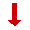# Why is it always 1089

This is the reasoning behind Always End With 1089.

We will need a little bit of algebra, but stick with it, it is not difficult.

Show the number as ABCReverse this to get CBARemember that A is Hundreds B is tens and C is units
Take CBA from ABC like this:Hundreds Tens Units A B C Subtract: C B A A−C 0 C−ANow here is the trick:
Subtract 1 Hundred, and add 9 Tens and 10 Ones
(−100, +90, +10 = 0, so won't change answer):

 Hundreds Tens Units A−1 B+9 C+10 Subtract: C B A A−1−C 9 10+C−ALast Step: Reverse the answer and